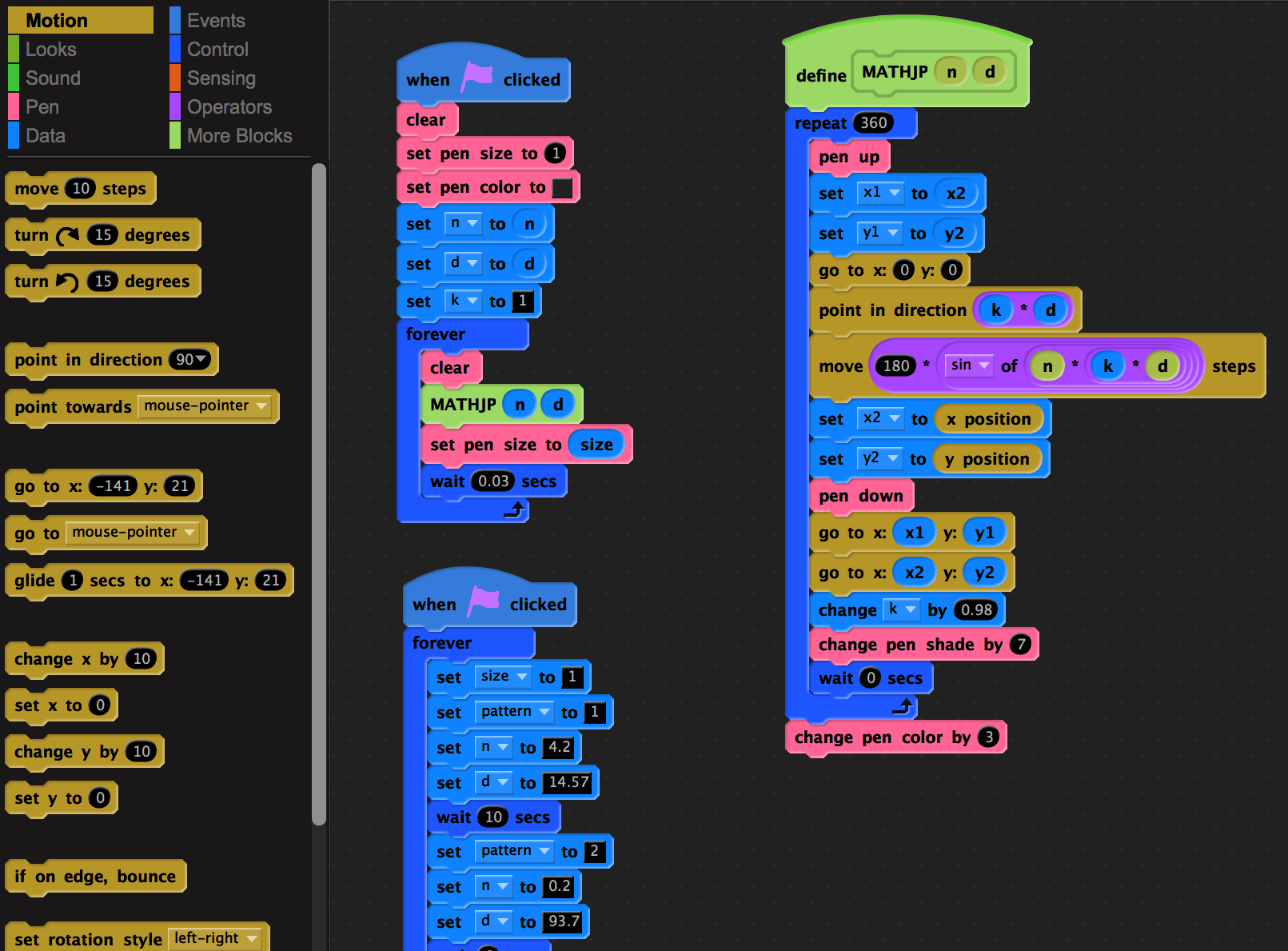## Maurer Roses

A rose is the polar curve, where the radius at angle t is sin(n t). Its parametrization in two dimensions is
``` r(t) =  <  sin(nt)  cos(t), sin(nt) sin(t) >
```
A Maurer rose is a polygonal curve connecting the points r(k a), where a is an angle. The pictures can be generated quickly with Mathematica. Here is one of the pictures you find on the Wikipedia page:
```n=6; d=71; M=500;    r[t_]:=Sin[n*t]{Cos[t],Sin[t]};
S1=ParametricPlot[r[t],{t,0,2Pi},PlotStyle->{Thickness[0.01],Red}];
S2=Graphics[{Blue,Line[Table[r[t*Pi*d/180],{t,0,M}]]}];Show[{S2,S1}]
```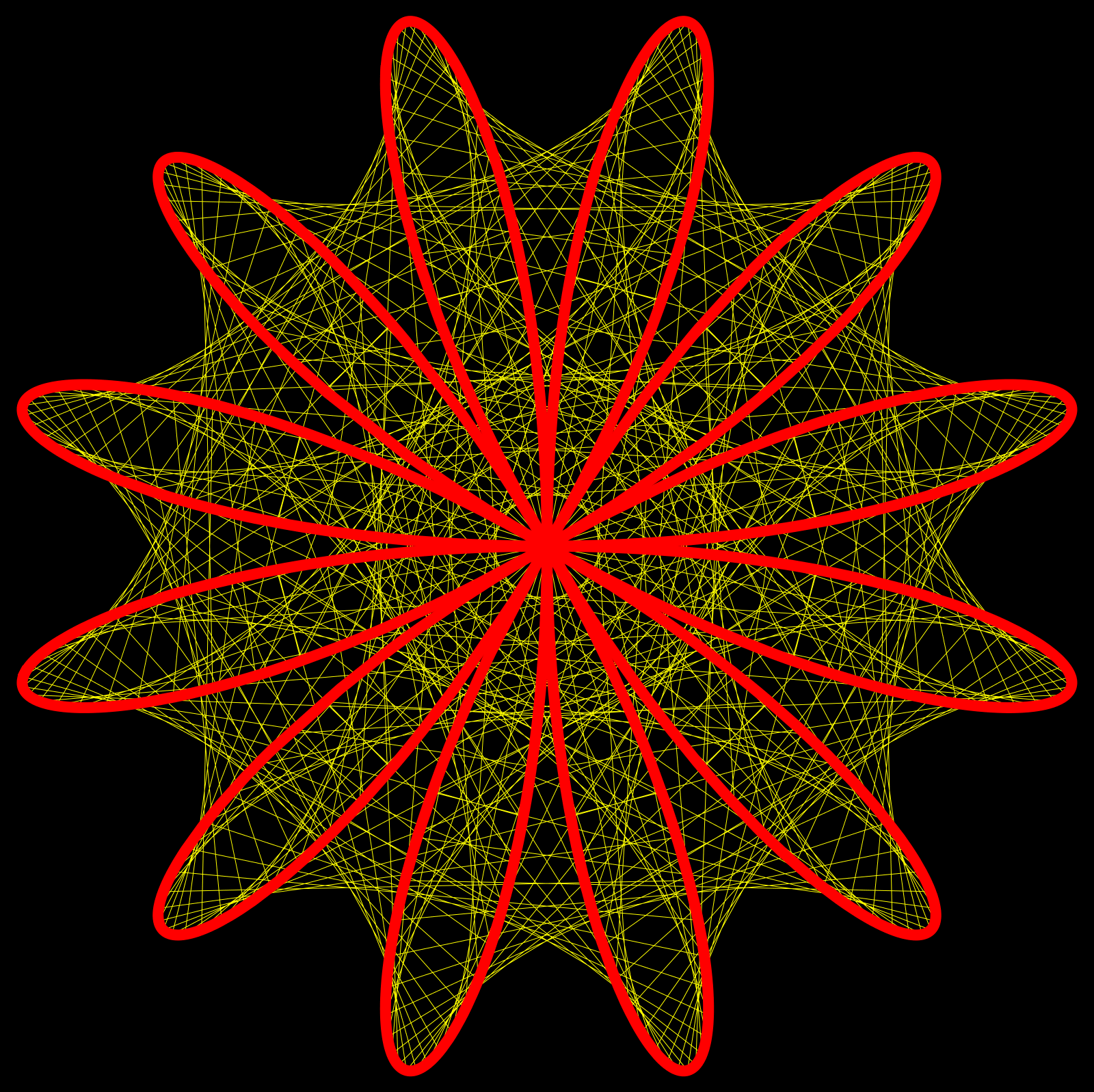Now, lets assume, you like a rose and want to see it in 3D. The adaptation to space is almost trivial. Just use "Tube" to produce curves and replace Graphics with Graphics3D. And maybe we like other colors and export it in a professional size (all graphics are vector graphics), maybe for a poster. Having an industrial strength software at hand, really helps here.
```n=2; d=31; M=100; r[t_]:=Sin[n*t]{Cos[t],Sin[t],0}; Show[{
Graphics3D[{Orange,Tube[Table[r[t], {t,0,2*Pi,0.01}],0.03]}],
Graphics3D[{Blue,Tube[Table[r[t*Pi*d/180],{t,0,M}],0.01]}]}]
```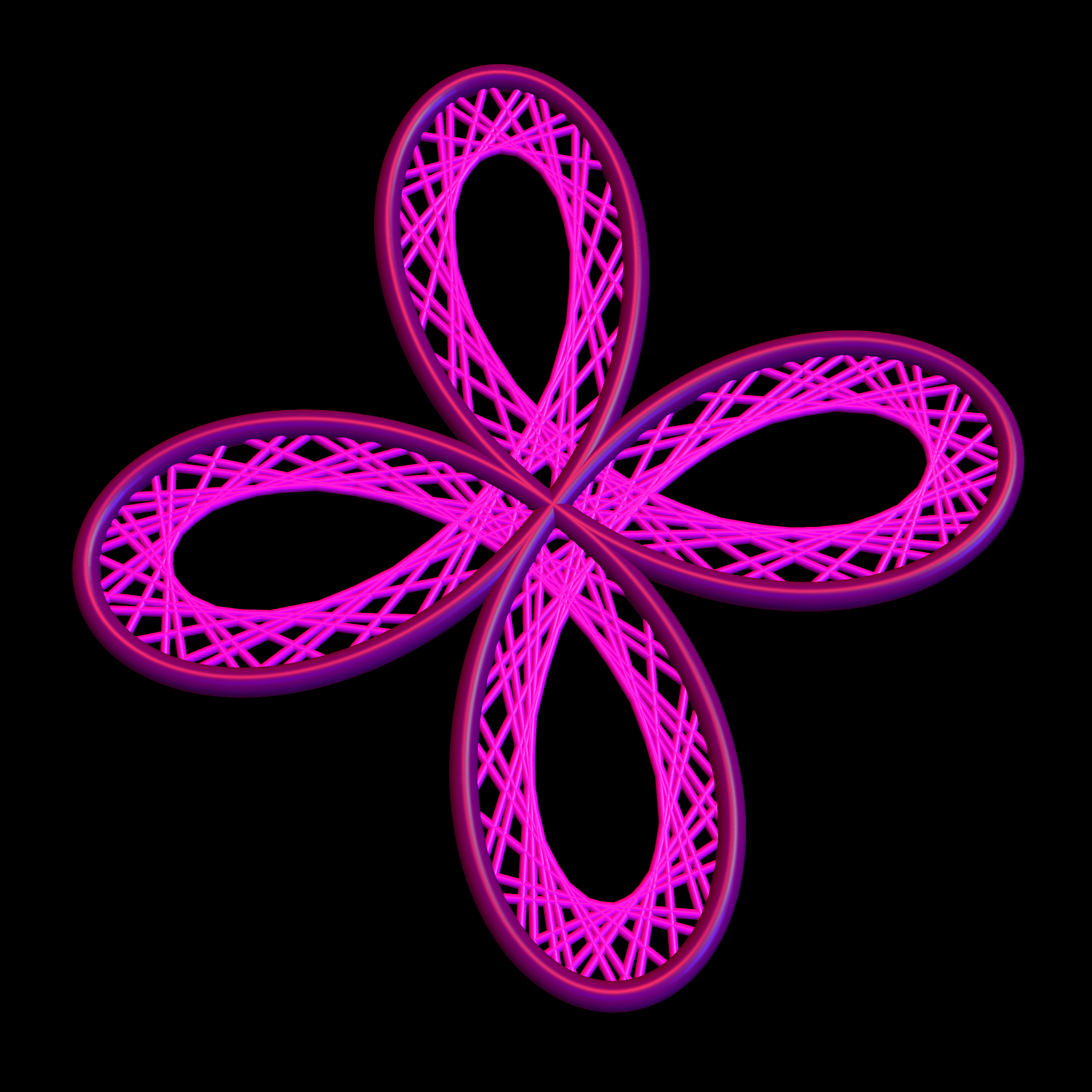Now, this can even be tweeted (including the picture, which soon will no more count against the 140 character limit: Assume we want to 3D print a rose, we just can export it as "STL":
```n = 3; d = 21; M = 50; r[t_]:=Sin[n*t] {Cos[t], Sin[t], 0};
S1=Graphics3D[Tube[Table[r[t], {t, 0, 2 Pi, 0.01}], 0.03]];
S2=Graphics3D[Tube[Table[r[t*Pi*d/180], {t, 0, M}], 0.01]];
Export["rose.stl",Show[{S1, S2}],"STL"]
```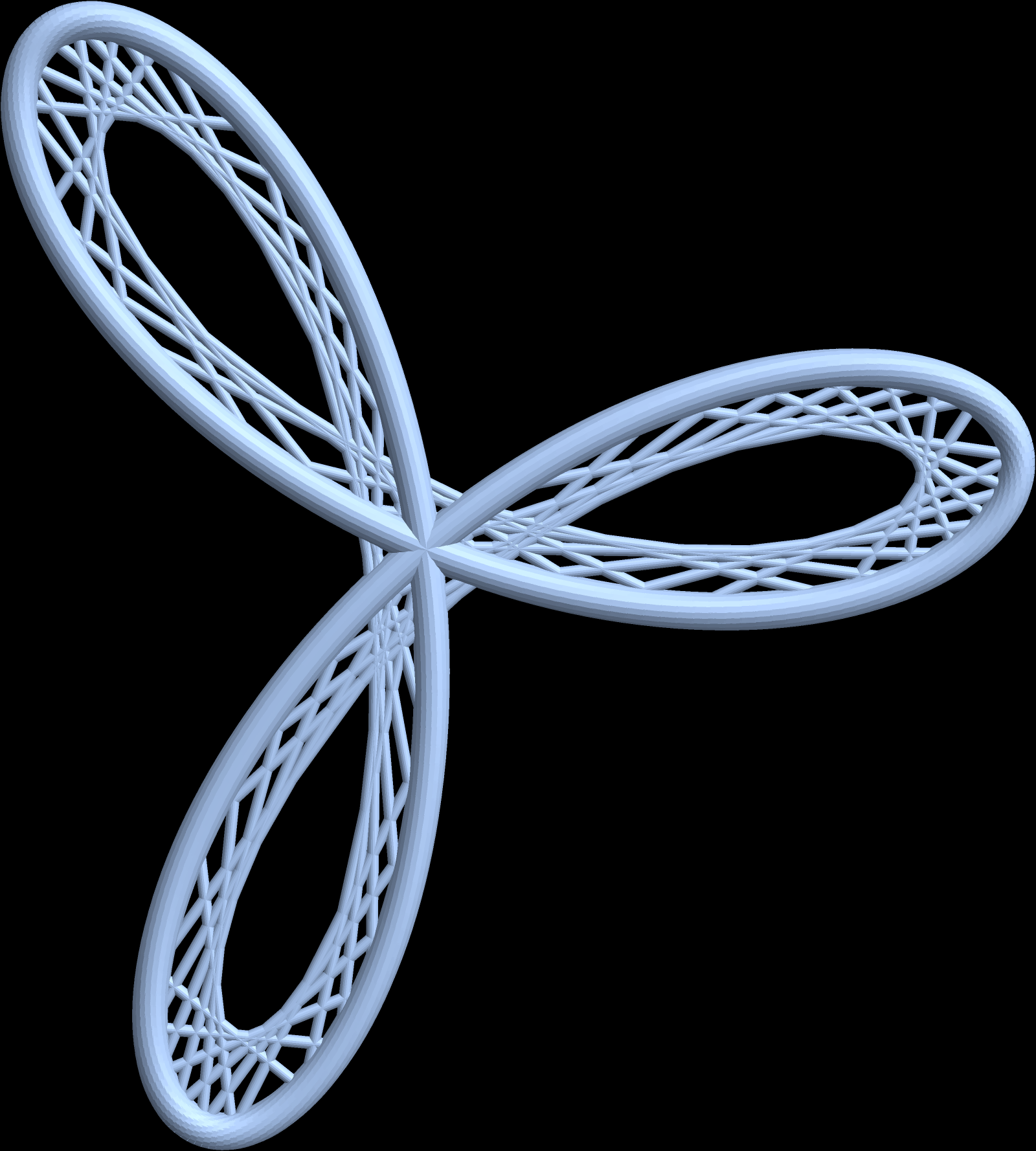By why stop here. Lets look at the sum of two roses. Math is great. Instead of adding numbers, we can also add curves and get a new curve! So, here is what we see if we so some arithmetic with roses:
``` r1(t) = < sin(n1 t) cos(t), sin(n1 t) sin(t),0 >
r2(t) = < sin(n2 t) cos(t), 0, sin(n2 t) sin(t)>
r(t)  = r1(t) + r2(t)
```
And here is the Mathematica code which produces the sum. And why use 5 lines of code for that if you can do it in 4 lines?
```n1=2; n2=3; d=31; M=100; r1[t_]:=Sin[n1*t]{Cos[t],Sin[t],0};
r2[t_]:=Sin[n2*t]{Cos[t],0,Sin[t]}; r[t_]:=r1[t]+r2[t]; Show[{
Graphics3D[{White,Tube[Table[r[t], {t, 0,2Pi,0.001}],0.03]}],
Graphics3D[{Blue, Tube[Table[r[t*Pi*d/180],{t,0,M}],0.01]}]}]
```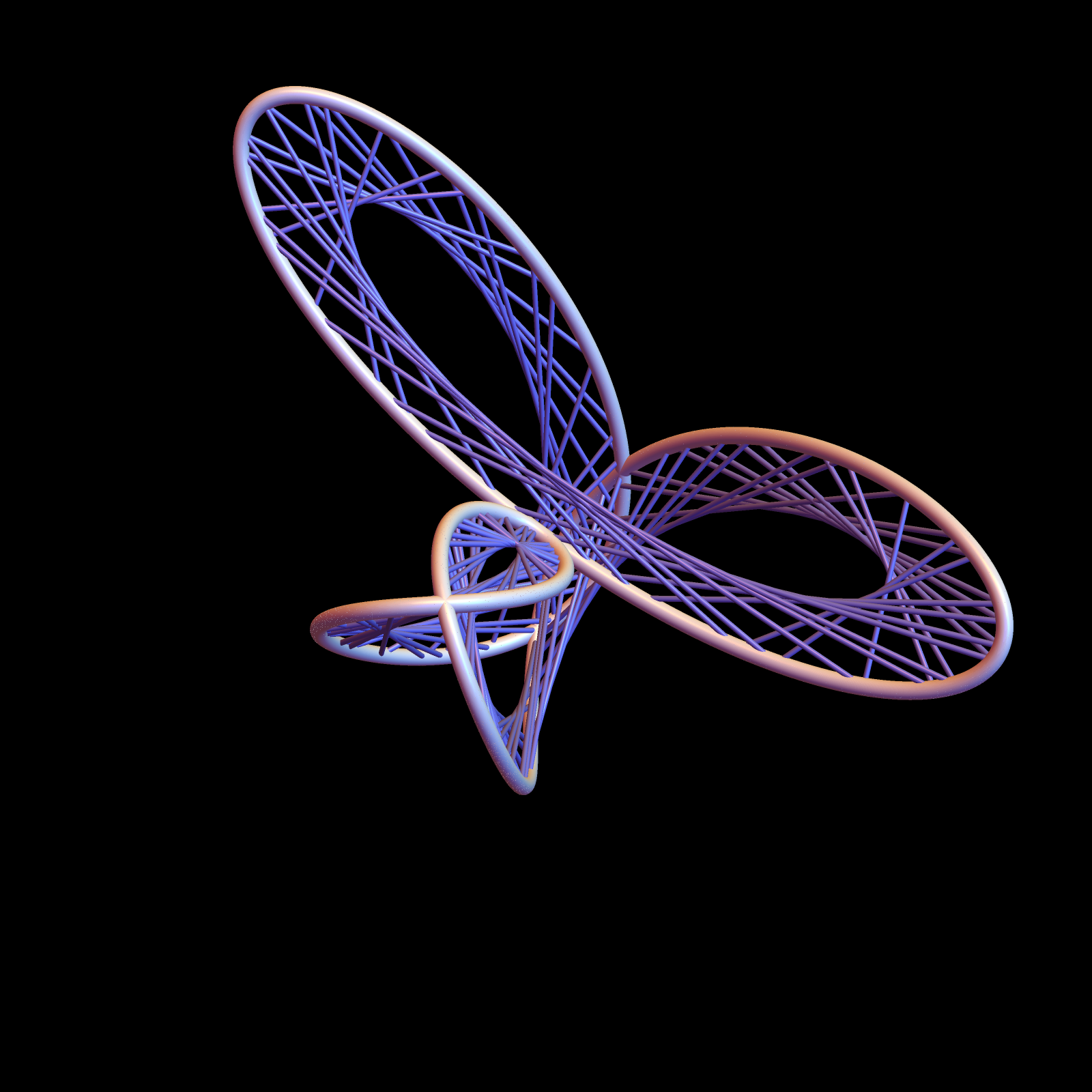Maurer roses are attractive. Here, you find a Scratch animation. I don't know how long the author of this scatch animation needed to write the code. Below is a screen shot containing about a third of the scratch code.